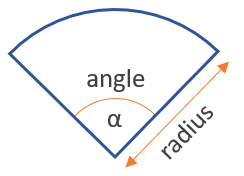# Area of a Sector Calculator

Use this calculator to easily calculate the area of a sector given its radius and angle.

°
Share calculator:

Embed this tool:
get code

## Area of a sector formula

The formula for the area of a sector is (angle / 360) x π x radius2. The figure below illustrates the measurement:As you can easily see, it is quite similar to that of a circle, but modified to account for the fact that a sector is just a part of a circle. Measuring the diameter is easier in many practical situations, so another convenient way to write the formula is (angle / 360) x π x (diameter / 2)2. The radius can be expressed as either degrees or radians, with our area of a sector calculator accepting only degrees for now (let us know if it would help you if it supported radians as well). π is, of course, the mathematical constant equal to about 3.14159.

## Area of a Sector calculation

You need to measure or know two things: the sector's radius and its angle. If the sector is a quadrant, then the angle is 90°. There are different tools for measuring angles, depending on your particular situation. A protractor can be useful in many cases.

After you have obtained the measurements, just apply the formula above or use our sector calculator as an easier and faster alternative.

## Example: find the area of a sector

As established, the only two measurements needed to calculate the area of a sector are its angle and radius. For example, if the angle is 45° and the radius 10 inches, the area is (45 / 360) x 3.14159 x 102 = 0.125 x 3.14159 x 100 = 39.27 square inches.

#### Cite this calculator & page

If you'd like to cite this online calculator resource and information as provided on the page, you can use the following citation:
Georgiev G.Z., "Area of a Sector Calculator", [online] Available at: https://www.gigacalculator.com/calculators/area-of-sector-calculator.php URL [Accessed Date: 27 Mar, 2023].